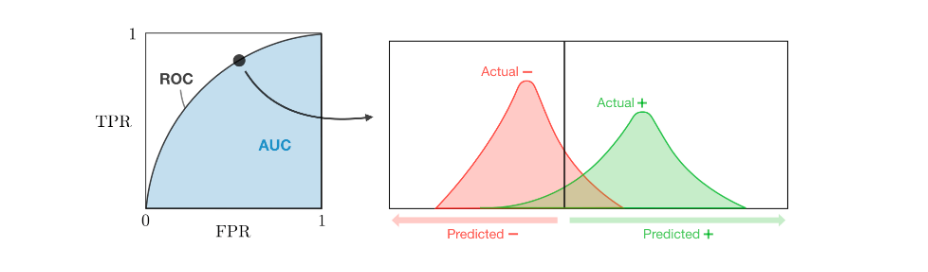# Machine Learning metrics

Original article was published by Zahra Elhamraoui on Deep Learning on Medium## Accuracy

The first and most fundamental classification metric is accuracy. Accuracy simply tells us how good is the classifier at sorting X s and Os. In other words, it is the number of true positives, added to the number of true negatives, and divided by the total number of data points.

The problem with classification accuracy is that it hides the detail you need to better understand the performance of your classification model.

## Precision

We might be also interested in how good is a classifier at avoiding false alarms. The metric used to calculate this is called precision. The precision of a classifier on a dataset is calculated by:

## Recall

If we are concerned about missing out and we want to catch as many true X s we can, we need a different metric called recall to measure our success. The recall is calculated by:

## F Score

This is a weighted average of the true positive rate (recall) and precision, This score is calculated according to:

ROC The receiver operating curve, also noted ROC, is the plot of TPR versus FPR by varying the threshold. These metrics are are summed up in the table below:

AUC The area under the receiving operating curve, also noted AUC or AUROC, is the area below the ROC as shown in the following figure:

# Regression metrics

Basic metrics Given a regression model ff, the following metrics are commonly used to assess the performance of the model:

Coefficient of determination The coefficient of determination, often noted R²R2 or r²r2, provides a measure of how well the observed outcomes are replicated by the model and is defined as follows:

Main metrics The following metrics are commonly used to assess the performance of regression models, by taking into account the number of variables nn that they take into consideration:

where L is the likelihood and σ2 is an estimate of the variance associated with each response.

# Conclusion

I was happy to share with you a very simple introduction into machine learning metrics with so simple words, that even people with no academic background would be able to understand it.

I hope I was able to clarify it a little to your machine learning, I will be uploading a lot of more explanation of algorithms because why not 🙂